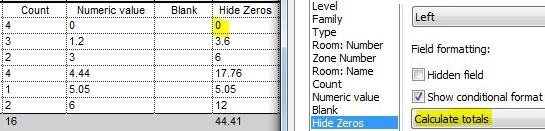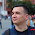## Friday, 9 August 2019

### Hide Zero Values in Multiple Item Rows in Revit SchedulesHide zero values in schedules (Previous post)

Following on from a previous post about how to Hide Zero Values in Revit Schedules, I have noticed that it does not work when you untick 'Itemise each Instance' (Multiple items per row) and you set the formatting to calculate totals - the zero returns.Calculate Totals - Zeros shown
In the example above, you probably would want to show the zeros when calculating totals of different numbers - but there are situations where you legitimately do not want to show the zero.  Here follows an example, and yet another workaround to solve that problem.

### Example of Workflow

A situation where you may not want to show the zeros might be when the field is a count of items.  As demonstrated in my previous "Count Dracula" post, it is not possible to include the system parameter 'Count' in a Schedule formula; nor is it possible to make a 'Count' value of zero - the workaround to that problem is to create your own 'MyCount' parameter, as described previously.

In this example,  items are to be listed in a schedule with consecutive room numbers - at this stage some of the rooms do not yet have any real items placed in them.  The schedule needs to show all the room numbers, even when not yet furnished.   In order for the rooms to appear in the schedule, a dummy item has to be placed in the room, but we do not want it to be formally counted.
This is where a 'MyCount' parameter is useful, as the dummy items can be forced to schedule with a count of zero.
• First a 'Schedule Count' parameter has to be created for the nominated categories
• Set the value to 1 for all items to be counted
• Set the value to zero for those not to be counted
• [NB. This could be a Y/N parameter, but that has complications - to be dealt with in another blog post]
• If it does not yet exist, create a 'Blank' calculated value, with no formula
(Refer to Hide Zeros)
• Then create a 'MyCount'  calculated value (Number or Integer)
• It can be given a formula of:
if(Schedule Count' = 0, Blank, Schedule Count)

• The schedule should list a 1 MyCount value for each item to schedule, and nothing for those Spare (dummy) items.

• Group the schedule by Room Number (no footers)
• Untick "Itemise every Instance"
• Notice that the MyCount total values are not totalling up
• Format 'MyCount' to calculate totals
•  Untick "Itemise every Instance"
• Aaaargh!   The zeros are back

### Workaround

In order to get the number of items in each room to total, you have to add a couple more calculated values - this is where it starts getting crazy!
• Add another Calculated Value 'MyPerc' or 'MyPercentage'
• Make it a Percentage (not a formula)
• Select the field 'MyCount' of which it is to show a percentage
• Select the Grouping title - 'Room Number' (rather than Grand Total) - so it only calculates a percentage of that Room sub-total

• Add another calculated value, with a formula:
if(MyCount/MyPerc = 0, Blank, MyCount/MyPerc)

• This will show the total count per Grouping (Room) in each row - which looks a bit crazy when each row is listed separately

• Untick 'Itemise every instance' and it looks better - and without the zeros
• Do not Calculate Totals for these two calculated values, otherwise it gives wrong totals

• Hide the intermediate calculated values, and rename the 'MyCountTotal' as desired

Now, if you actually want to show Grand Totals for the Item Count, that is another story . . . .
• You would probably need to create another schedule that shows 'MyCount' (which gives the correct total), but collapse it down to one row (no sorting/grouping; no grant total) - and align it on a sheet with the column for MyCountTotal.
• That would also enable you to have bold text for the total
• However, I am not going to try that here - you are on your own.
[Edit.  A comment from Kovyljan has pointed out that the last method above can be simplified by making the percentage calculate on 'Schedule Count' instead of MyCount - refer to ps Update on Hide Zeros ]

For more information on this technique, refer to an earlier post on using Totals in Formulas *

* Thanks to Martijn de Riet of BIMForce who initially posted on his blog a similar solution to another scheduling problem in Revit

1.2.